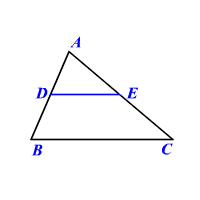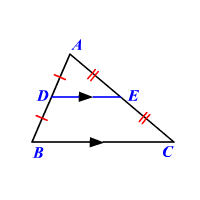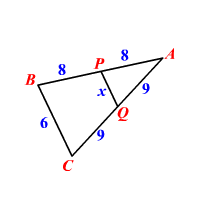# Triangle Midsegment Theorem

## Midsegment

A midsegment of a triangle is a segment that connects the midpoints of two sides of a triangle.In the figure $D$ is the midpoint of $\stackrel{¯}{AB}$ and $E$ is the midpoint of $\stackrel{¯}{AC}$ .

So, $\stackrel{¯}{DE}$ is a midsegment.

## The Triangle Midsegment Theorem

A midsegment connecting two sides of a triangle is parallel to the third side and is half as long.If $AD=DB\text{\hspace{0.17em}}\text{\hspace{0.17em}}\text{and}\text{\hspace{0.17em}}\text{\hspace{0.17em}}AE=EC,$

then $\stackrel{¯}{DE}\parallel \stackrel{¯}{BC}$ and $DE=\frac{1}{2}BC$ .

Example :

Find the value of $x$ .Here $P$ is the midpoint of $AB$ , and $Q$ is the midpoint of $BC$ . So, $\stackrel{¯}{PQ}$ is a midsegment.

Therefore by the Triangle Midsegment Theorem,

$PQ=\frac{1}{2}BC$

Substitute.

$\begin{array}{l}x=\frac{1}{2}\cdot 6\\ \text{\hspace{0.17em}}\text{\hspace{0.17em}}\text{\hspace{0.17em}}\text{\hspace{0.17em}}=3\end{array}$

The value of $x$ is $3$ .